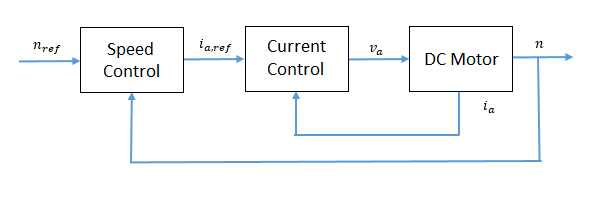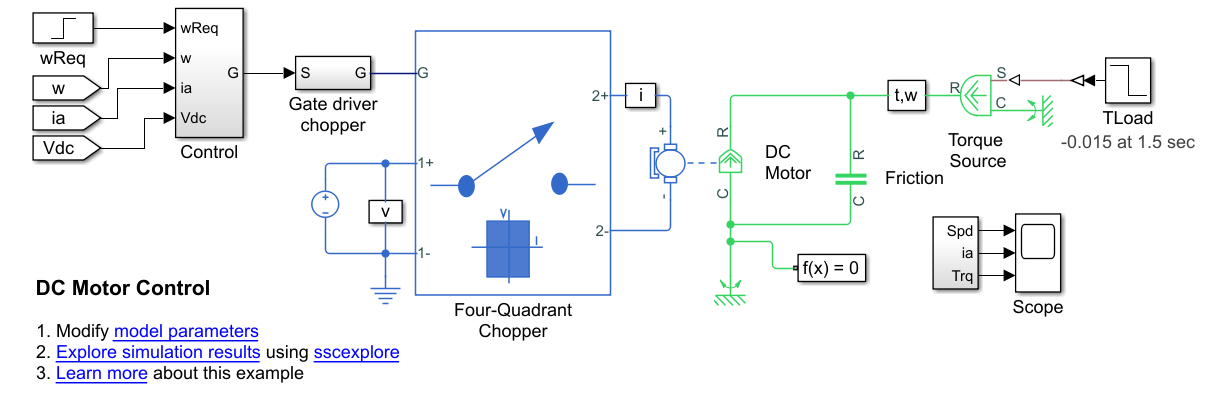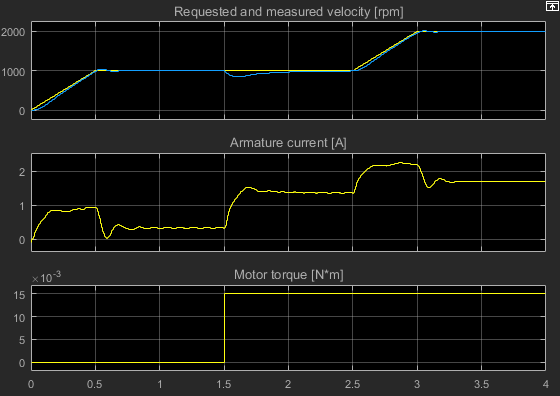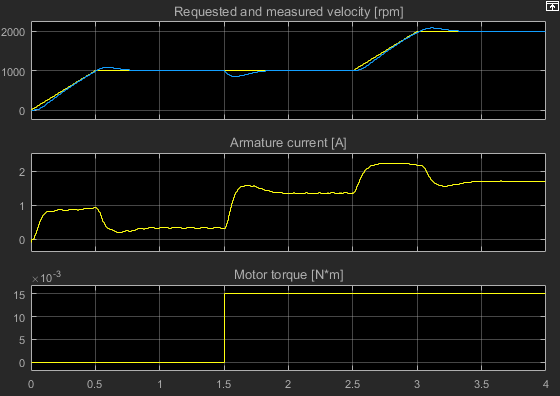## Tune an Electric Drive

This example shows how to tune an electric drive using a cascade control structure.

The figure shows a feedback control loop that uses a cascade control structure. The outer speed-control loop is slower acting than the inner current-control loop.### Equations for PI Tuning Using the Pole Placement Method

To satisfy the required control performance for a simple discrete plant model, Gf (z-1), use a closed loop PI control system GPI(z-1). The transient performance can be expressed in terms of the overshoot. The overshoot decreases relative to the damping factor:

`$\sigma ={e}^{\frac{-\pi \xi }{\sqrt{1-{\xi }^{2}}}}$`

where,

• σ is overshoot.

• ξ is the damping factor.

The response time, tr, depends on the damping and the natural frequency, ωn, such that:

• If ξ < 0.7,

`${t}_{r}\cong \frac{4}{{\omega }_{n}\xi }.$`
• If ξ ≥ 0.7,

`${t}_{r}\cong \frac{6\xi }{{\omega }_{n}}.$`

The general workflow for designing a PI controller for a first-order system is:

1. Discretize the plant model using the zero-order hold (ZOH) discretization method. That is, given that the first-order equation representing the plant is

`$G\left(s\right)=\frac{{K}_{m}}{{T}_{m}s+1},$`

where,

• Km is the first-order gain.

• Tm is time constant of the first-order system.

Setting

`$s=\frac{1-{z}^{-1}}{{z}^{-1}{T}_{s}},$`

yields the discrete plant model,

`$G\left({z}^{-1}\right)=\frac{{K}_{m}\left(\frac{{T}_{s}}{{T}_{m}}\right){z}^{-1}}{1+\left(\frac{{T}_{s}-{T}_{m}}{{T}_{m}}\right){z}^{-1}}=\frac{{b}_{1}{z}^{-1}}{1+{a}_{1}{z}^{-1}},$`

whereTs is sample time for the discrete-time controller.

2. Write a discrete-time representation for the PI controller using the same transform. For

`${G}_{PI}\left(s\right)={K}_{P}+{K}_{I}\left(\frac{1}{s}\right),$`

setting

`$s=\frac{1-{z}^{-1}}{{z}^{-1}{T}_{s}},$`

yields the discrete controller model,

`${G}_{PI}\left({z}^{-1}\right)=\frac{{K}_{P}+\left({K}_{I}{T}_{s}-{K}_{P}\right){z}^{-1}}{1-{z}^{-1}}=\frac{{q}_{0}+{q}_{1}{z}^{-1}}{1-{z}^{-1}}.$`

Combining the discrete equations for the plant and the controller yields the closed loop transfer function for the system,

`${G}_{0}\left({z}^{-1}\right)=\frac{{q}_{0}{b}_{1}{z}^{-1}+{q}_{1}{b}_{1}{z}^{-2}}{1+\left({a}_{1}-1+{q}_{0}{b}_{1}\right){z}^{-1}+\left(-{a}_{1}+{q}_{1}{b}_{1}\right){z}^{-2}},$`

The denominator of the transfer function is the characteristic polynomial. That is,

`${P}_{c0}\left({z}^{-1}\right)=1+\left({a}_{1}-1+{q}_{0}{b}_{1}\right){z}^{-1}+\left(-{a}_{1}+{q}_{1}{b}_{1}\right){z}^{-2}.$`

3. The characteristic polynomial for achieving the required performance is defined as

`${P}_{cd}\left({z}^{-1}\right)=1+{\alpha }_{1}{z}^{-1}+{\alpha }_{2}{z}^{-2},$`

where,

• ${\alpha }_{1}=-2{e}^{-\xi {\omega }_{n}{T}_{s}}\mathrm{cos}\left({\omega }_{n}{T}_{s}\sqrt{1-{\xi }^{2}}\right).$

• ${\alpha }_{2}={e}^{-2\xi {\omega }_{n}{T}_{s}}.$

4. To determine the controller parameters, set the characteristic polynomial for the system equal to the characteristic polynomial for the required performance. If

`${P}_{c0}\left({z}^{-1}\right)={P}_{cd}\left({z}^{-1}\right),$`

then

`${\alpha }_{1}={a}_{1}-1+{q}_{0}{b}_{1}$`

and

`${\alpha }_{2}=-{a}_{1}+{q}_{1}{b}_{1}.$`

Solving for q0 and q1 yields

`${q}_{0}=\frac{{\alpha }_{1}-{a}_{1}+1}{{b}_{1}}$`

and

`${q}_{1}=\frac{{\alpha }_{2}+{a}_{1}}{{b}_{1}}.$`

Therefore, the general equations for the proportional and integral control parameters for the first-order system are

`${K}_{P}={q}_{0}$`

and

`${K}_{I}=\frac{{q}_{1}+{K}_{p}}{{T}_{s}}.$`

### Equations for DC Motor Controller Tuning

Assuming that, for the system in the example model, Kb = Kt, the simplified mathematical equations for voltage and torque of the DC motor are

`${v}_{a}={L}_{a}\frac{d{i}_{a}}{dt}+{R}_{a}{i}_{a}+{K}_{b}\omega$`

and

`${T}_{e}={J}_{m}\frac{d\omega }{dt}+{B}_{m}\omega +{T}_{load}={K}_{b}{i}_{a},$`

where:

• va is the armature voltage.

• ia is the armature current.

• La is the armature inductance.

• Ra is the armature resistance.

• ω is the rotor angular velocity

• Te is the motor torque.

• Jm is the rotor moment of inertia.

• Bmis the viscous friction coefficient.

• Kb is a constant of proportionality.

To tune the current controller, assume that the model is linear, that is, that the back electromotive force, as represented by Kbω, is negligible. This assumption allows for an approximation of the plant model using this first-order Laplace equation:

`${G}_{i}\left(s\right)=\frac{\frac{1}{{R}_{a}}}{\left(\frac{{L}_{a}}{{R}_{a}}\right)s+1}.$`

Given the system requirements, you can now solve for KP and KI. The requirements for the current controller in the example model are:

• Sample time, Ts= 1 ms.

• Overshoot, σ = 5%.

• Response time, tr = 0.11 s.

Therefore, the proportional and integral parameters for the current controller are:

• ${K}_{P}=7.7099.$

• ${K}_{I}=455.1491.$

To tune the speed controller, approximate the plant model with a simple model. First assume that the inner loop is much faster than the outer loop. Also assume that there is no steady-state error. These assumptions allow for the use a first-order system by considering a transfer function of 1 for the inner current loop.

To output rotational velocity in revolutions per minute, the transfer function is multiplied by a factor of 30/π. To take as control input the armature current instead of the motor torque, the transfer function is multiplied by the proportionality constant, Kb. The resulting approximation for the outer-loop plant model is

`${G}_{n}\left(s\right)=\frac{\frac{30{K}_{b}}{\pi {B}_{m}}}{\left(\frac{{J}_{m}}{{B}_{m}}\right)s+1}.$`

The speed controller has the same sample time and overshoot requirements as the current controller, but the response time is slower, such that:

• Sample time Ts= 1 ms.

• Overshoot σ = 5%.

• Response time tr = 0.50 s.

Therefore, the proportional and integral parameters for the speed controller are:

• ${K}_{P}=0.0045$

• ${K}_{I}=0.0405$

### Tune the Electric Drive in the Example Model

1. Explore the components of the DC motor and the cascaded controller.

1. Open the model. At the MATLAB® command prompt, enter

```model = 'ee_dc_motor_control' open_system(model)```2. The Control subsystem contains the model of the cascaded control system built using blocks from the Simulink® library.3. The Four Quadrant Chopper block represents a four-quadrant DC-DC chopper that contains two bridge arms, each of which has two IGBT (Ideal, Switching) blocks. When the input voltage exceeds the threshold of `0.5` V, the IGBT (Ideal, Switching) blocks behave like linear diodes with a forward-voltage of ```0.8 V``` and a resistance of `1e-4` ohm. When the threshold voltage is not exceeded, the IGBT (Ideal, Switching) blocks act like linear resistors with an off-state conductance of `1e-5` 1/ohm.

2. Simulate the model.

`sim(model)`

3. View the results. Open the Scope block.At 1.5 seconds, there is a load torque that results in a steady-state error.

4. Tune the DC motor controller. The `ee_getDCMotorFirstOrderPIParams` function calculates the proportional gain, KP, and the integral gain, KI, for the first-order system in this example.

The function syntax is `[Kp, Ki] = getParamPI(Km,Tm,Ts,sigma,tr)`.

The input arguments for the function are the system parameters and the requirements for the controller:

• `Km` is the first-order gain.

• `Tm` is the time constant of the first-order system.

• `Ts` is the sample time for the discrete-time controller.

• `sigma` is the desired maximum overshoot, σ.

• `tr` is the desired response time.

1. To examine the equations in the function, enter

`edit ee_getDCMotorFirstOrderPIParams`

2. To calculate the controller parameters using the function, save these system parameters to the workspace:

```Ra=4.67; % [Ohm] La=170e-3; % [H] Bm=47.3e-6; % [N*m/(rad/s)] Jm=42.6e-6; % [Kg*m^2] Kb=14.7e-3; % [V/(rad/s)] Tsc=1e-3; % [s] ```

3. Calculate the parameters for tuning the current controller as a function of the parameters and requirements for the inner controller:

• `Km` = `1/Ra`.

• `Tm` = `La/Ra`.

• `Ts` = `Tsc`.

• `sigma` = `0.05`.

• `Tr` = `0.11`.

`[Kp_i, Ki_i] = ee_getDCMotorFirstOrderPIParams(1/Ra,La/Ra,Tsc,0.05,0.11)`

```Kp_i = 7.7099 Ki_i = 455.1491```

The gain parameters for the current controller are saved to the workspace.

4. Calculate the parameters for tuning the speed controller based on the parameters and requirements for the outer controller:

• `Km` = `Kb*(30/pi)`.

• `Tm` = `Jm/Ra`.

• `Ts` = `Tsc`.

• `sigma` = `0.05`.

• `Tr` = `0.5`.

`[Kp_n, Ki_n] = ee_getDCMotorFirstOrderPIParams((Kb*(30/pi))/Bm,Jm/Bm,Tsc,0.05,0.5)`

```Kp_n = 0.0045 Ki_n = 0.0405```

The gain parameters for the speed controller are saved to the workspace.

5. Simulate the model using the saved gain parameters for the speed and controllers.

`sim(model)`

6. View the results. Open the Scope block.There is slightly more overshoot, however, the controller responds much faster to the load torque change.